2.2. Exponents:

How many zeros are there in a crore and in thousand crores?

Observe the following few quotes from  Yuddha kanda in Ramayana . It’s period is said to be at least 4000BC.

Shatam shata sahasranam koti mahurmahishana: ||1||

Meaning:    100  *  100 * 1000     = koti(crore)

Shatam koti  sahasranam shanka ityabhidhiyate ||2||

Meaning:    100  * koti * 1000     = Shankha

Shatam shankha   sahasranam mahashankha itismrata: || 3||

Meaning:    100  * shankha * 1000    = Mahashankha

The verses go on till Mahougha which is 1 followed by 62 zeros (= 1062)

This shows that during those days itself, they were familiar with zero and well conversant with the decimal number system.

In this topic we learn easy method of representation and multiplication of large numbers

We know 16 = 2*2*2*2 (the number 2 is multiplied 4 times)

Therefore we say 16 is equal to 4th power of 2 and write 16 = 24. We say 16 is equal to ‘2 raised to the power of 4

16 = 4*4 = 42 (We also say 16 is equal to ‘4 raised to the power of 2’)

Like the way we factorize numbers we can also factorize algebraic expressions.

For example x3= x*x*x

We say x3  is ‘x raised to the power of 3’

This method of easy representation of x*x*x by x3 is called ‘exponential notation’.

In general:

xn   = x *x*x* …. n times

Here x is called base and n is called exponent or ‘index’

Base Exponent = Number

Note a = a1

2.2 Problem 1: Express 1331 to base of 11

Solution:

We know the factors of 1331 are 11, 11, 111331 = 11*11*11 = 113

Let us find the product of 25 and  2325 *23 = (2*2*2*2*2)*(2*2*2) = 28

Did you notice that 8 =5+3?

1. From the above observation we arrive at the first law (Product Law) of exponents:

If x is a non zero real number and m and n are numbers then xm  *xn   = x(m+n)

2.2 Problem 2: Evaluate a14 *b32 * a4 *b16

Solution:

a14 *b32 * a4 *b16

= (a14 * a4 )*(b32 * b16)  ( By rearranging terms)

= (a14+4)*(b32+16) (By first law)

=a18 *b48

Let us divide 25 by 2325 /23 = (2*2*2*2*2)/(2*2*2) =  2*2=22

Similarly 23 /25 = (2*2*2)/ (2*2*2*2*2) = 1/(2*2) = 1/(22)

23 /23 = (2*2*2)/(2*2*2) = 1

2. From the above observation we arrive at the second law (Quotient Law) of exponents:

If x is a non zero real number and m and n are numbers with m>n then xm  /xn   = x(m-n)

If x is a non zero real number and m and n are numbers with m<n then xm  /xn   = 1/(x(n-m)  )

By definition, for any x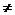0

1) xm = 1/( x-m)

2) x-m = 1/ ( xm)

3) If n is a positive integer and a0 then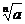= a1/n

4) If a0 and n0  then am/n=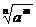Observe:

x0 =1 (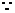1 = xm  /xm   = x(m-m)  )

2.2 Problem 3: Write equivalents for 10-5  and 2/m-1

Solution:

10-5 = 1/105

2/m-1= 2/(1/m1) = 2m1 =2m

2.2 Problem 4: Evaluate xa+b /xb-c

Solution:

xa+b /xb-c

= xa+b /1/(x-(b-c)) (By definition)

= xa+b *x-(b-c)

= xa+b+(-(b-c))  (By Second law)

= xa+b-b+c(-(b-c) = -b+c)

= xa+c

Let us find the product of 52 , 52 and 5252 *52*52= (5*5)*(5*5)*(5*5) = 56

We can write this also as

52 *52*52 = (52)3 = 52*3

3. From the above observation we arrive at the third law (Power law) of exponents:

If x is a non zero real number and m and n are  numbers then (xm  )n   = xmn

2.2 Problem 5 : Evaluate [{(x2)2}2]2

Solution:

(x2)2= x4

{(x2)2}2 = {x4}2 = x8

[{(x2)2}2]2 =  [x8]2= x16

Exercise: Verify the answer by expanding the terms individually

Let us evaluate (2*5)3

(2*5)3 = (2*5)*(2*5)*(2*5) (By definition)

= (2*2*2)*(5*5*5) (By Grouping  all 2 and 5 together)

= (2)3*(5)3

4. From the above observation we arrive at the fourth law of exponents:

If x and y are non zero real numbers and m is a number then (x*y)m    = (xm)* (ym)

2.2 Problem 6: Simplify (5x-3 y-2)3

Solution:

(5x-3 y-2)3

= (5)3 *(x-3)3*(y-2)3 ( By fourth law)

= 53* x-9* y-6             (By third law)

= 53/( x9* y6)           (By definition)

Exercise: Verify the answer by expanding the terms individually

2.2 Problem 7: Simplify (3x-2 y)-1

Solution:

(3x-2 y)-1

= (3) -1*( x-2)-1 *(y)-1  --àBy fourth law

= (3) -1* x+2 *y-1 ----à By third law

= x2 /3*y--à By definition

Verify the answer by expanding the terms individually

Let us evaluate  (2*5)3

(2/5)3 = (2/5)*(2/5)*(2/5) (By definition)

= (2*2*2)/(5*5*5)  ( Group all 2 and 5 together)

= (2)3/(5)3

4. From the above observation we arrive at the fifth law of exponents:

If x and y are non zero real numbers and m is a number then (x/y)m    = (xm)/ (ym)

Observations:

Since (-1)2 = (-1)*(-1) =+1 and (-1)3 = (-1)*(-1)*(-1) = -1 we note:

1. If m is an even number then (-a)m = (-1)m *am = am

2. If m is an odd number then (-a)m == (-1)m *am = -am

Proof :

1.    If m is even then m is of the form 2n ( n=1,2,3..)(-1)m = (-1)2n = ((-1)2 )n       ----à 3rd law

= 1n = 1

2.    If m is odd then m is of the form 2n+1 ( n=0,1,2,3..)(-1)m = (-1)2n+1 = (-1)2n *(-1)1      ----à 2nd Law.

= 1n *-1              ----à(proved in the previous case )

=  -1

2.2 Problem 8 : Simplify (am/an)p*(an/ap)m*(ap/am)n

Solution :

(am/an)p

= (am)p/(an)p   (By fifth law)

= amp/ anp          (By  third law)(am/an)p*(an/ap)m*(ap/am)n

= (amp/ anp)* (anm/ apm)* (apn/ amn) (By expanding other terms)

= (amp* anm* apn)/ (anp*apm*amn)(Note numerator and denominator are same)

=1

2.2 Problem 9 : Simplify (a4b-5/ a2b-4)-3

Solution:

Let us first, simplify the term

(a4b-5/ a2b-4)

= (a4/ a2) * (b-5/ b-4)

= (a2/ b) (By second law - (a4/ a2) = (a4-2) = a2, (b-5/ b-4) = (b-5-(-4)) = b-5+4= b-1= 1/b )

Now let us take the given problem

(a4b-5/ a2b-4)-3

= (a2/ b)-3        (Substitute the simplified term for (a4b-5/ a2b-4)

= (a2)-3/ (b)-3 (By third law)

=a-6/b-3

= b3/a6

Alternate method: Let us solve this problem in another way

(a4b-5/ a2b-4)-3

= (a-12b+15/ a-6b+12)        (By third law)

=(a-12/ a-6)* (b15/ b12)   ( By grouping terms)

=(a-12* a6)* (b15* b- 12) ( By definition x -m = 1/( xm)

=(a-12+6)* (b15-12)         ( By first   law)

=a-6*b3

= b3/a6

2.2 Summary of learning

 No Points to remember 1 By definition xn = x*x*x*x – n times 2 Base Exponent = Number 3 By definition  x0 =1 4 By definition   x - m = 1/( xm) 5 First law :xm  *xn   = x(m+n) 6 Second law xm  /xn   = x(m-n) 7 Third law (xm  )n   = xmn 8 Fourth law (x*y)m    = (xm)* (ym) 9 Fifth law (x/y)m    = (xm)/ (ym)

If x is a positive rational number and m(=p/q) a positive rational exponent, then we define  xp/q as the ‘qth root’ of xp or alternatively

xp/q as the ‘pth power ’ of x1/q.

i.e.  xp/q = (xp)1/q=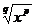= (x1/q)p= (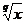)p

Note: (r/s)-p/q =(s/r)p/q

The form x1/m  is called ‘exponential form’ and if m>0, then the form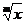is called ‘radical form’. The sign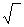is called the radical sign andis called ‘radical’. The number m is called ‘index’ of the radical and x is called the ‘radicand’.

Note, index is always a positive number .

2.2 Problem 10: If 1960 = 2a5b7c calculate the value of = 2-a7b5-c

Solution:

1960  =  2*2*2*5*7*7=  235172a=3, b=1 and c=2

Hence

2-a =1/8 and  5-c =1/25

Thus

2-a7b5-c

= (1/8)*7*(1/25) = 7/200

2.2 Problem 11: Simplify {(8x3)/125y3}2/3

Solution:

We know:

8x3 = (2x)3 and 125y3 = (5y)3(8x3)/125y3

= (2x/5y)3{(8x3)/125y3}2/3

= {(2x/5y)3}2/3

= (2x/5y)3*2/3

= (2x/5y)2

= 4x2/25y2

2.2 Problem 12: Find x if 3x-1 = 9*34

Solution:

9 = 329*34 = 32*34 = 36

Since

3x-1 = 9*34 = 36,

x-1 = 6x=7

Verification:

Substitute x=7 in the given problem and expand the terms to note that the answer = 729.